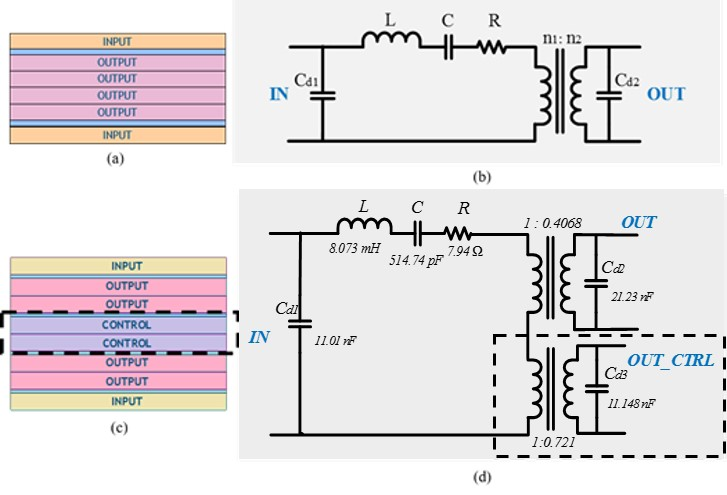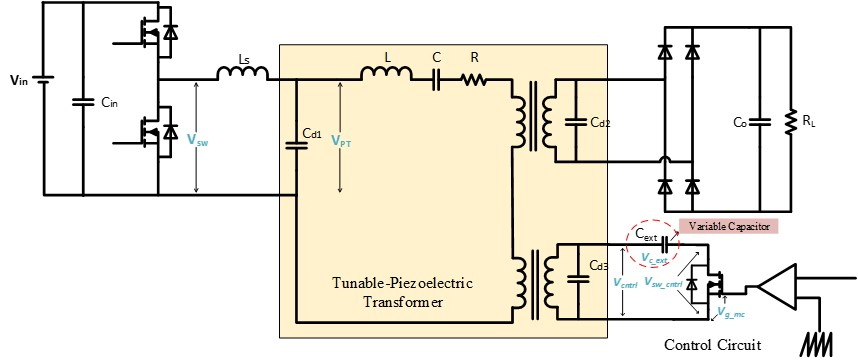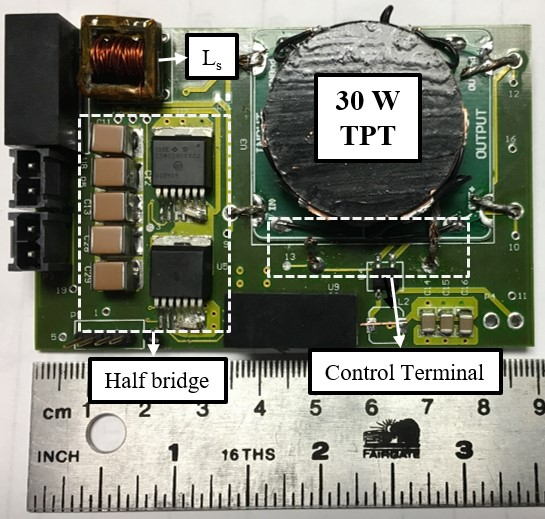LIBRARY

# New Tunable Piezoelectric Transformers and their Application in dc-dc ConvertersFig. 1. (a) Structure of layers in a standard Transoner® radial PT and (b) its electrical equivalent circuit. (c) and (d) show the structure of a Tunable PT with control sec-tion sandwiched in between the input and output layers.
Recently, radial Piezoelectric Transformer (PT) structures, such as the so-called Transoner® PT, have been developed and are used for ac-dc and dc-dc converter applications. Just like resonant converters, most of the converters using PTs regulate the output voltage by changing the switching frequency of the inverter bridge (variable frequency modulation control). This requires isolation in the feedback circuit between the primary and secondary, which adds to the weight and complexity of the converter. This paper discusses the basic operation of a new Tunable Piezoelectric Transformer (TPT) unit. It proposes a TPT-based dc-dc converter rated at 30 W, 120:55 V DC, and a fixed frequency control scheme to regulate the output voltage under load and input voltage variations.

The proposed Tunable PT uses the same structure as the radial PT, however, an additional piezoelectric section (control layer) is sandwiched between the existing primary and secondary layers, as shown in Fig. 1. A change in the control capacitance modifies the primary series capacitance of the control layer, thus changing the resonant frequency of the piezoelectric transformer structure. To implement this, a control scheme has been proposed, as shown in Fig. 2 with an external cap whose value can be regulated from 2 nF - 100 nF using duty cycle control.

The proposed design is implemented on hardware using a 30W TPT developed by Micromechatronics, Inc., PA and is shown in Fig. 3. With the nominal voltage of 220 V DC, an operating frequency of 82.5 kHz and a nominal load of 105 &Omega, the output voltage is regulated at 55 V using the constant frequency control.

This paper introduces the concept behind new tunable piezoelectric transformers and demonstrates their implementation in the design of dc-dc converters. The proposed control scheme provides a way to regulate the output voltage using a fixed frequency control and without isolation in the control circuit.Fig. 2. Electrical schematic for dc-dc converter design using tunable PT.Fig. 3. A dc-dc converter board developed using a 30W Tunable PT.